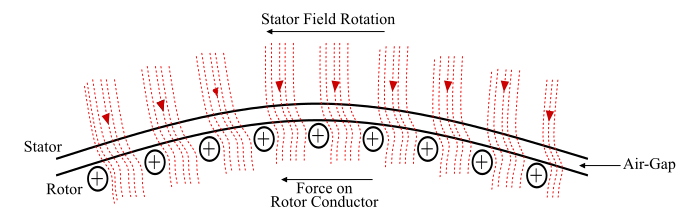A 3-phase induction motor is an electromechanical energy conversion device which converts 3-phase input electrical power into output mechanical power.A 3-phase induction motor consists of a stator and a rotor. The stator carries a 3-phase stator winding while the rotor carries a short-circuited winding called rotor winding. The stator winding is supplied from a 3-phase supply. The rotor winding drives its voltage and power from the stator winding through electromagnetic induction and hence the name.

## Working Principle of a 3-Phase Induction Motor

The working principle of a 3-phase induction motor can be explained by considering a portion of it as follows−• When the 3-phase stator winding is fed from a balanced 3-phase supply, a rotating magnetic field (RMF) is produced in the motor. This RMF rotates around the stator at synchronous speed which is given by,

$$\mathrm{Synchronous\:Speed, \:­Øæü_­Øæå = \frac{120­Øæō}{­Øæā}}$$

• The RMF passes through the air gap and cuts the rotor conductors, which as yet are stationary. Due to the relative motion between the RMF and the stationary rotor conductors, EMFs are induced in the rotor conductors. As the rotor circuit is closed with short-circuit so currents start flowing in the rotor conductors.
• Since the current carrying rotor conductors are placed in the magnetic field produced by the stator winding. As a result, the rotor conductors experience mechanical force. The sum of the mechanical forces on all the rotor conductors produce a torque which moves the rotor in the same direction as the rotating magnetic field. Hence, in such a way the three phase input electric power is converted into output mechanical power in a 3-phase induction motor.
• Also, according to Lenz’s law, the rotor should move in the direction of the stator field, i.e., the direction of rotor currents would be such that they tend to oppose the cause producing them. Here, the cause producing the rotor currents is the relative speed between the RMF and the rotor conductors. Thus to reduce this relative speed, the rotor starts running in the same direction as that of the RMF.

## Advantages of Three Phase Induction Motor

Following are the chief advantages of a 3-phase induction motor −

• It has simple and rugged construction.
• It requires less maintenance.
• It has high efficiency and good power factor.
• It is less expensive.
• It has self-starting torque.

## Disadvantages of Three Phase Induction Motor

The disadvantages of a 3-phase induction motor are given as follows −

• The 3-phase induction motors are constant speed motors; hence their speed control is very difficult.
• 3-phase induction motors have poor starting torque and high inrush currents (about 4 to 8 times of the rated current).
• They always operate under lagging power factor and during light loads, they operate at very worst power factor (about 0.3 to 0.5 lagging).